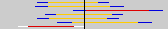# Instructions

A "Begin" button will appear on the left when the applet is finished loading. This may take a minute or two depending on the speed of your internet connection and computer. Please be patient. If no begin button appears, it is probably because your browser does not support Java 1.1. Click here for information on how to get a browser that does.

Starting the Applet and setting the conditions
Press the "Begin" button to start the applet in another window.

This applet simulates sampling from a population with a mean of 50 and a standard deviation of 10. For each sample, the 95% and 99% confidence intervals on the mean are computed based on the sample mean and sample standard deviation. The intervals for the various samples are displayed by horizontal lines as shown below. The first two lines represent samples for which the 95% confidence interval contains the population mean of 50. The 95% confidence interval is orange and the 99% confidence interval is blue. In the third line, the 95% confidence interval does not contain the population mean; it is shown in red. In the seventh and last line shown below, the 99% interval does not contain the population mean; it is shown in white.Specify whether you want the sample size to be 10, 15, or 20 scores and click the "Sample" button. Then, 100 samples of the specified sample size will be taken and the resulting 100 confidence intervals will be plotted.

The cumulative number of confidence intervals containing and not containing the population parameters is tallied.

In this simulation, you know that the population mean is 50. Naturally, a researcher will not know the value of the population mean and will not know whether a confidence interval he or she computes contains the population mean.